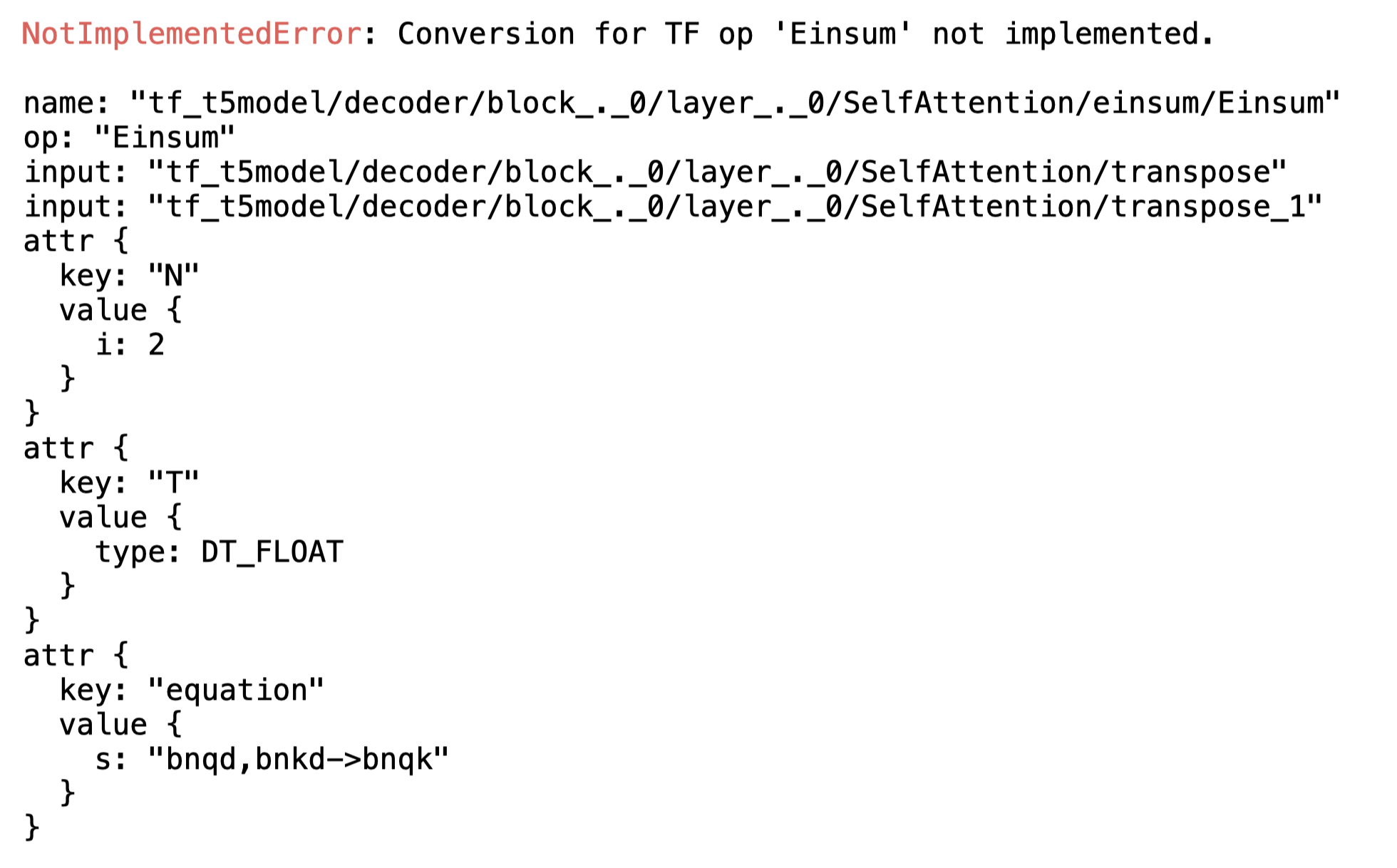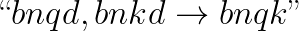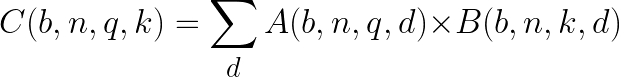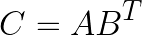## coremltools

Use Core ML to integrate machine learning models into your app. Core ML provides a unified representation for all models. Your app uses Core ML APIs and user data to make predictions, and to train or fine-tune models, all on the user’s device.

Core ML optimizes on-device performance by leveraging the CPU, GPU, and Neural Engine while minimizing its memory footprint and power consumption. Running a model strictly on the user’s device removes any need for a network connection, which helps keep the user’s data private and your app responsive.

# Composite Operators

As machine learning continually evolves, new operations are regularly added to source frameworks such as TensorFlow and PyTorch. While converting a model to Core ML, you may encounter an unsupported operation.

In most cases, you can handle unsupported operations by using composite operators, which you can construct using the existing MIL operations. For an overview of MIL operations, see Model Intermediate Language.

All of the operations in the MIL Builder class are at your disposal to construct a composite operator. The following example defines a mb.matmul operation using the ML Builder to construct a composite operator. It converts the T5 Model, available in the Transformers library.

### 📘Install Transformers

You may need to first install Transformers version 2.10.0.

# Import and convert the pre-trained model

1. Add the import statement and load the pre-trained model:
from transformers import TFT5Model

model = TFT5Model.from_pretrained('t5-small')

1. The returned object is an instance of a tf.keras model, which you can pass directly into the coremltools converter:
import coremltools as ct

mlmodel = ct.convert(model)

1. To simulate a scenario in which coremltools lacks support for an operation, disable the Einsum operation needed to convert the model:
from coremltools.converters.mil.frontend.tensorflow.tf_op_registry import _TF_OPS_REGISTRY

del _TF_OPS_REGISTRY["Einsum"]

1. Run the conversion. The following error occurs, indicating an unsupported TensorFlow operation:# Decompose into existing MIL operators

The TensorFlow documentation on Einsum refers to Einstein summation notation. You can use this notation to represent a variety of tensor operations such as reduce_sum, transpose, and trace, using a string. Einsum is usually a complicated operation, but with this example you don't need to know all the possible cases, just the particular notation that this model uses.

The error trace shows that the model uses the following notation for Einsum:The above notation translates into the following mathematical expression:While the above may look complicated, it is effectively a batched matrix multiplication with a transpose on the second input:You can decompose this operation with existing MIL operators. In fact, MIL supports this operation directly. To write a composite for this operation, follow these steps:

1. Import MIL builder and a decorator:
from coremltools.converters.mil import Builder as mb

from coremltools.converters.mil import register_tf_op

1. Define a function with the same name as the TensorFlow operation. For this example, this is Einsum. To define the function, grab inputs and define a matmul operation using the MIL builder:
@register_tf_op
def Einsum(context, node):

assert node.attr['equation'] == 'bnqd,bnkd->bnqk'

a = context[node.inputs]
b = context[node.inputs]

x = mb.matmul(x=a, y=b, transpose_x=False,
transpose_y=True, name=node.name)



### 📘Register the function

Be sure to decorate this function to register it with the converter, as shown in the above code for TensorFlow (@register_tf_op). The decoration ensures that the user-defined function will be invoked whenever an Einsum operation is encountered during the conversion. The corresponding decorator for PyTorch is @register_torch_op.

1. With composite operation for Einsum defined, call the Core ML converter again and print mlmodel. This verifies that the conversion is completed, and implies that the unsupported operation error is resolved:
mlmodel = ct.convert(model)
print(mlmodel)


# Using Composite Ops with PyTorch Conversion

For PyTorch, the following import statement can be used:

# example pytorch composite op

from coremltools.converters.mil.frontend.torch.torch_op_registry import
_TORCH_OPS_REGISTRY, register_torch_op
from coremltools.converters.mil.frontend.torch.ops import _get_inputs
from coremltools.converters.mil import Builder as mb

del _TORCH_OPS_REGISTRY["selu"] # only required if over-writing an existing translation

@register_torch_op
def selu(context, node):
x = _get_inputs(context, node, expected=1)
x = mb.elu(x=x, alpha=1.6732632423543772)
x = mb.mul(x=x, y=1.0507009873554805, name=node.name)

model = ct.convert(....)


# More examples

For more examples, see the translation code for the supported TensorFlow ops and PyTorch ops.Updated 7 months ago

# Composite Operators

### Suggested Edits are limited on API Reference Pages

You can only suggest edits to Markdown body content, but not to the API spec.